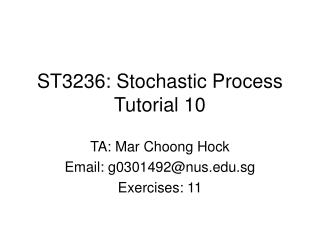Download PresentationST3236: Stochastic Process Tutorial 10

Loading in 2 Seconds...

# ST3236: Stochastic Process Tutorial 10 - PowerPoint PPT Presentation

ST3236: Stochastic Process Tutorial 10. TA: Mar Choong Hock Email: g0301492@nus.edu.sg Exercises: 11. Question 1. For i = 1 , … , n. Let {X i ( t ) : t > 0 } be independent Poisson processes, each with the same parameter  . Find the distribution of the first timeI am the owner, or an agent authorized to act on behalf of the owner, of the copyrighted work described.
Download Presentation## ST3236: Stochastic Process Tutorial 10

An Image/Link below is provided (as is) to download presentation

Download Policy: Content on the Website is provided to you AS IS for your information and personal use and may not be sold / licensed / shared on other websites without getting consent from its author.While downloading, if for some reason you are not able to download a presentation, the publisher may have deleted the file from their server.

- - - - - - - - - - - - - - - - - - - - - - - - - - E N D - - - - - - - - - - - - - - - - - - - - - - - - - -
Presentation Transcript
1. ST3236: Stochastic ProcessTutorial 10 TA: Mar Choong Hock Email: g0301492@nus.edu.sg Exercises: 11

2. Question 1 For i = 1, … , n. Let {Xi(t) : t > 0} be independent Poisson processes, each with the same parameter . Find the distribution of the first time that at least one event has occurred in every process.

3. Question 1 Note: You can give either the c.d.f. or the p.d.f. as the answer for this case, the c.d.f. is F(t) = 1 - {1 - exp(-tl)}n

4. Question 2 Let {X(t) : t 0} be a Poisson process of rate . Suppose it is known that X(t) = n. For n = 1, 2, … , determine the mean of the first arrival time W1 and Wn. Note: The Wn here is conditioned on X(t) = n. This is different from tutorial 9.

5. Question 2 Let Y1, … , Ynbe IID and uniformly distributed on (0, t]. Then W1 has the same distribution as Y(1) and Wnhas the same distribution as Y(n). Note: Yi is a random variable that represents the position of customer i, on the time axis (measured w.r.t t = 0), after placing the customer on the axis (uniform distribution). It is not necessary the ith arrived customer. By definition, Y(1) = min {Y1, Y2, …, Yn}, that is, it is the first arrived customer.

6. Question 2 Then W1 has the same distribution as Y(1) and Wn has the same distribution as Y(n) (since it is the maximum of all Yi it represents the last arrived customer). The distribution of Y(1) is

7. Question 2

8. Question 2

9. Question 3 Let {X(t) : t 0} be a Poisson process of rate . Suppose it is known that X(t) = 2. Determine the mean W1W2, the product of the first two arrival times

10. Question 3 Let Y1 and Y2 be IID and distributed uniformly on (0; t]. We have E(Y1) = E(Y2) = t/2 Let Y(1) and Y(2) be the order statistics of Y1 and Y2. Then (W1, W2) have the same distribution as (Y(1), Y(2)). Because Y(1)Y(2) = Y1Y2 Thus E(W1W2) = E(Y1Y2) = E(Y1)E(Y2) = t2/4

11. Question 4 • Customers arrive at a certain facility according to a • Poisson process of rate . Suppose that it is know • that five customers arrived in the first t hours. • Determine the mean total waiting time • E{W1 + … +W5 | X(t) = 5} • (b) Determine the mean total waiting time • E{W1 + … +W5+W6| X(t) = 5}

12. Question 4 Let Y1, …, Y5 be IID and distributed uniformly on (0, t]. We have E(Y1) = … = E(Y5) = t/2 Let Y(1), …, Y(5) be the order statistics. Then (W1, … ,W5) have the same distribution as (Y(1), … , Y(5)). Because Y(1) + … + Y(5) = Y1 + … + Y5

13. Question 4a Thus E(W1 + … +W5 | X(t) = 5) = E(Y1 + … + Y5) = E(Y1) + … + E(Y5) = 5t/2

14. Question 4b E{W1 + … +W5 +W6|X(t) = 5} = E{W1 + … +W5 | X(t) = 5 }+ E {W6| X(t) = 5} = 5t/2 + (t +1/) (See lecture notes for the calculation of E{W6 | X(t) = 5} )